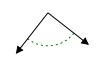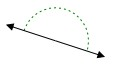# Acute, obtuse, and right angles

We measure angles in degrees. Angles can also be measured in other units like radians. One complete rotation around a point is 360 degrees or 2 pi radians. We use a little circle ° following the number to mean degrees. For example, 60° means 60 degrees. A full circle is 360°; half a circle is 180° and quarter a circle in 90°.

We often measure angles using a protractor. The normal protractor measures 0° to 180°

An acute angle is defined as an angle that is more than 0° but less than 90° in measure.

A right angle is defined as an angle that is 90° in measure.

An obtuse angle is defined as an angle that is between 90° and 180° in measure.

Classify each angle as acute, obtuse, right or straight### Solution

Step 1:

Given angle is equal to 90°.

Step 2:

So, the given angle is a right angle.

Classify each angle as acute, obtuse, right or straight### Solution

Step 1:

Given angle is equal to 180°.

Step 2:

So, the given angle is a straight angle